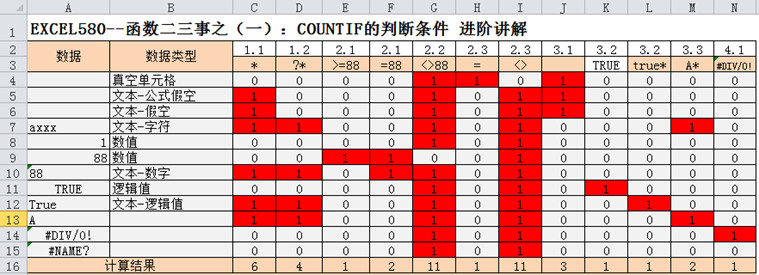# EXCEL880–COUNTIF的判断条件 进阶讲解 通配符 模糊匹配

2015年10月11日00:15:28 评论 3,828 views

COUNTIF 俗称条件计数，来计算符合条件的单元格个数，接受参数为（单元格区域，条件） 基本用法可参考帮助1.通配符 "*","?"

*表示0-N个文本字符,?表示一个文本字符

1.1 COUNTIF(A4:A15,"*")=6符合条件单元格为A5,A6,A7,A10,A12,A13

1.2 COUNTIF(A4:A15,"?*")=4符合条件单元格为A7,A10,A12,A13

"*"代表了所有的文本包含(""),如果想求出不含空文本的所有文本单元格个数时,使用"?*",是一个不错的选择

2.比较运算

2.1 等于运算和其他比较运算的一些不同

COUNTIF(A4:A15,"=88") =2符合条件单元格为A9,A10

2.2 不等于运算

COUNTIF(A4:A15,"<>88")=11符合条件单元格为A4,A5,A6,A7,A8,A10,A11,A12,A13,A14,A15

<>比较数值时要慎用 确保没有文本型数值，实在没办法去除用 COUNTA(B3:B12)-COUNTIF(A4:A15,"=88")

2.3 真空问题

COUNTIF(A4:A15,"=")=11符合条件单元格为A4

COUNTIF(A4:A15,"<>")=11符合条件单元格为A5,A6,A7,A8,A9,A10,A11,A12,A13,A14,A15

3.文本与逻辑值

3.1 COUNTIF(A4:A15,"")=3符合条件单元格为A4,A5,A6

3.2 COUNTIF(A4:A15,TRUE)=1符合条件单元格为A11

COUNTIF(A4:A15,"true*")=1符合条件单元格为A12

3.3 COUNTIF文本比较

COUNTIF(A4:A15,"A*")=2符合条件单元格为A7,A13

4.错误值

COUNTIF(A4:A15,1/0)1符合条件单元格为A14

COUNTIF(A4:A15,"#value!")

**ps:以上的判断条件在sumif中是通用的,是完全一样的.

• 郑广学老师微信号
• EXCEL880B
•• 我的微信公众号
• EXCEL880
•